26 Feb 2016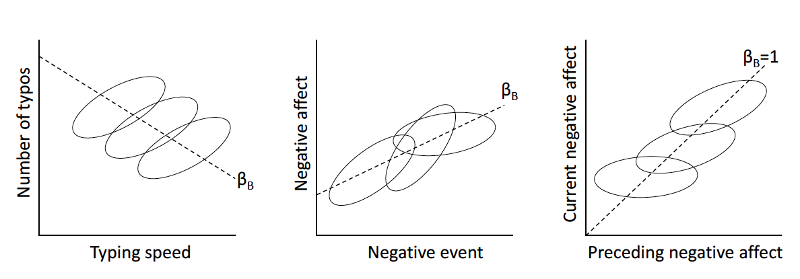• Hamaker, E. L., & Grasman, R. P. P. P. (2014). To center or not to center? Investigating inertia with a multilevel autoregressive model. Frontiers in Psychology, 5, 1492. http://doi.org/10.3389/fpsyg.2014.01492

## Experience Sampling Method (ESM)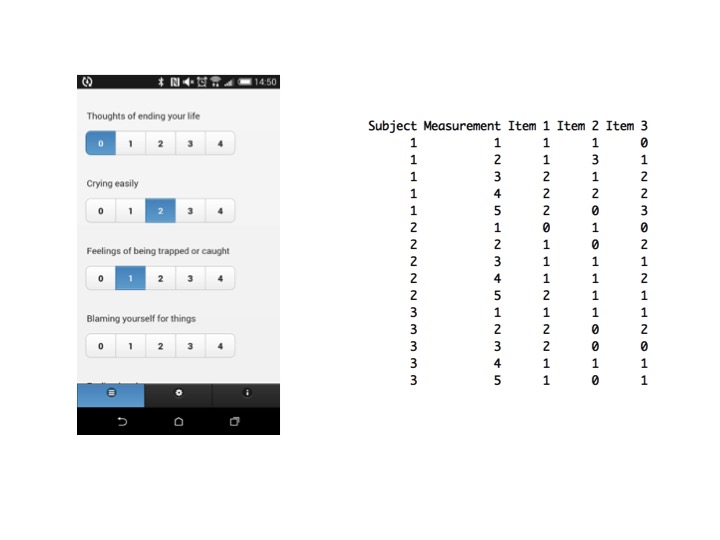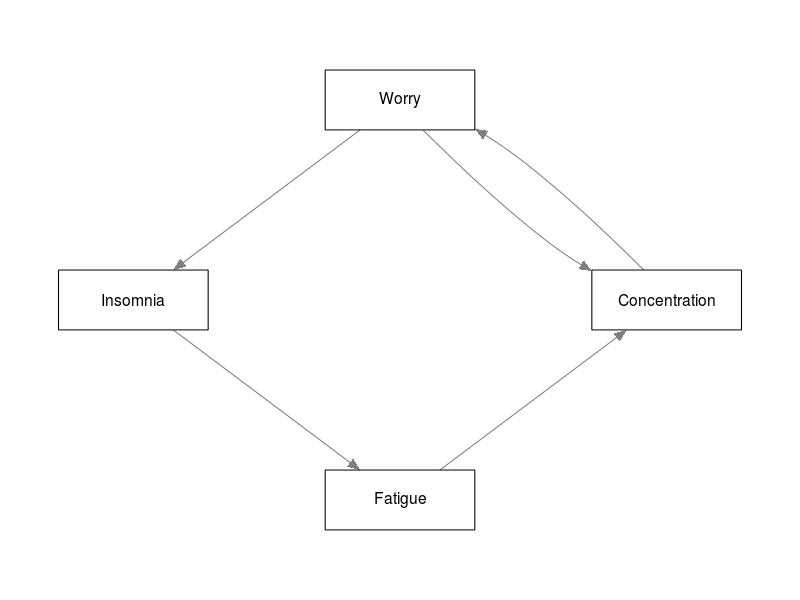## The Dynamics of Psychology

• Psychological constructs can be conceptualized as dynamical systems, featuring complex emergent behavior:
• Correlated responses
• Stable "traits"
• Phase transitions
• Individual Differences
• These systems can be portrayed as networks

## Outline

• Introduction Vector Auto-regression
• Problem 1: $$n=1$$ and limited observations
• Graphical VAR
• Problem 2: $$n>1$$
• Multi-level VAR
• Problem 3: Measurement error
• State-space models
• Even more problems

## Vector Auto-Regression (VAR)

• Regress a vector of variables from a single subject on the previous time point
• Assume multivariate normality
• Assume errors are correlated
• This leads to three things to estimate:
• A vector of intercepts
• A matrix encoding a temporal network
• A matrix encoding a contemporaneous network
• Can be estimated using lm() or any least squares regression method

For a single subject: \begin{aligned} \pmb{y}_t &= \pmb{\tau} + \pmb{B} \pmb{y}_{t-1} + \pmb{\varepsilon}_t \\ \pmb{\varepsilon}_t &\sim N\left( \pmb{0}, \pmb{\Theta} \right). \end{aligned} $$\pmb{B}$$ encodes a directed temporal network and $$\pmb{\Theta}$$ and undirected contemporaneous network

## Vector Auto-Regression (VAR)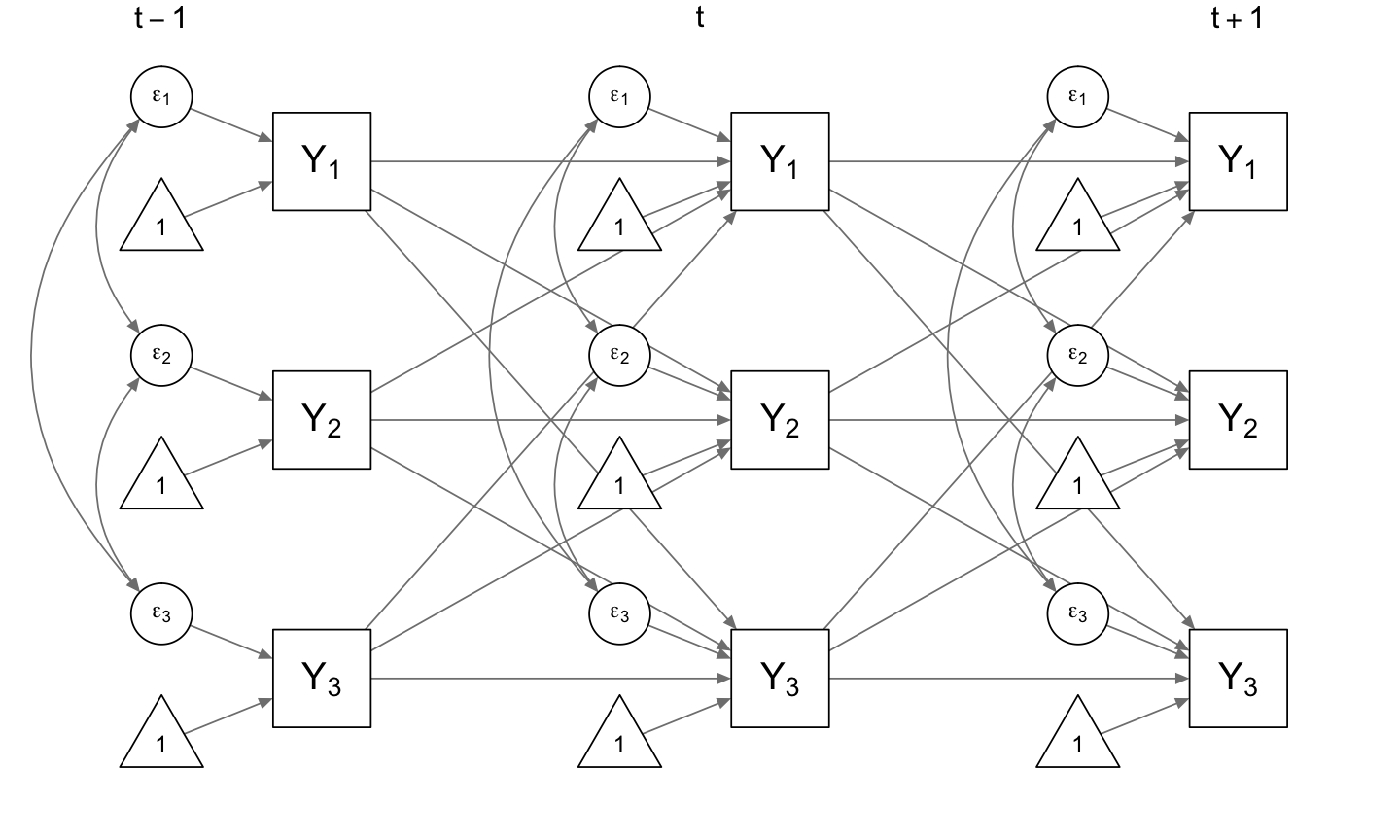## Vector Auto-Regression (VAR)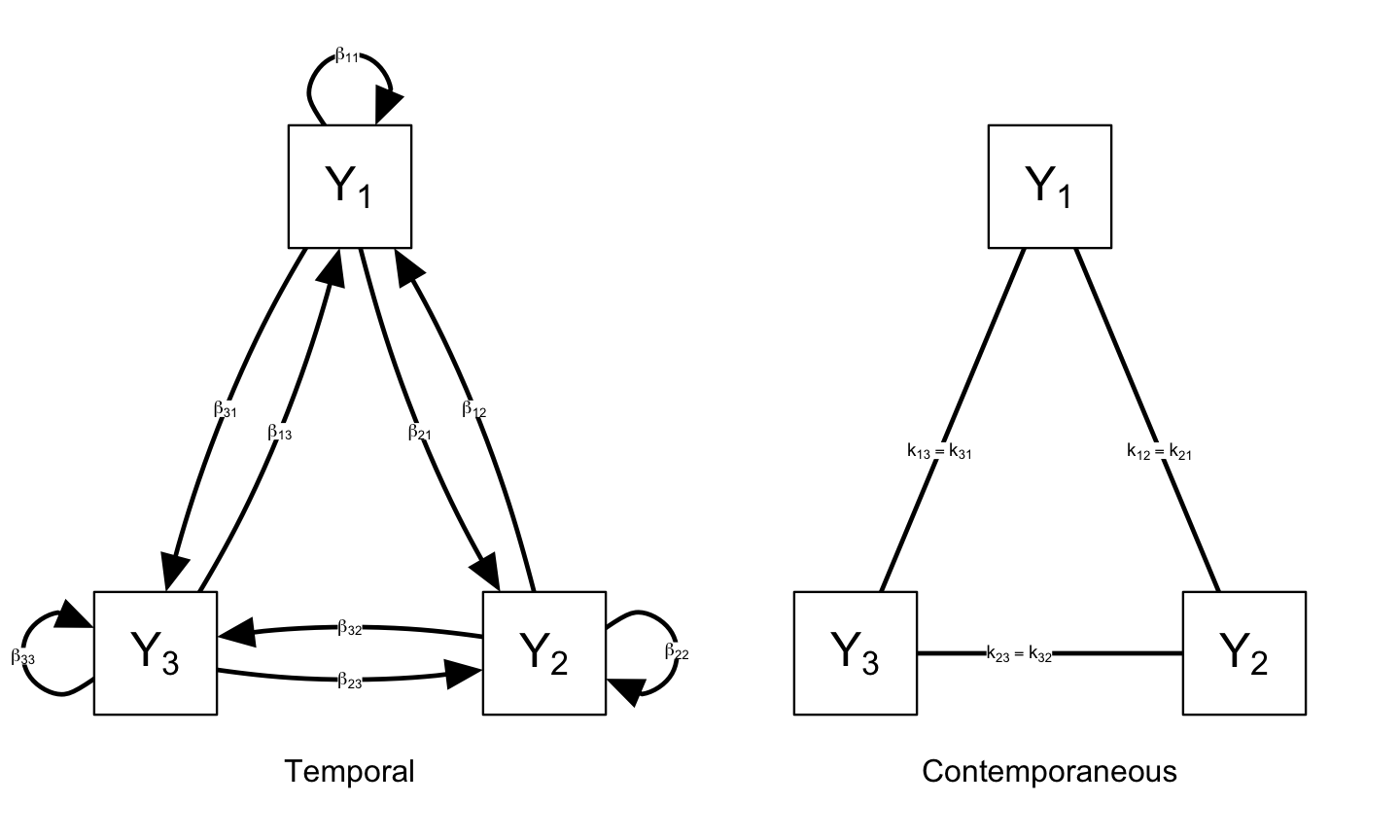With $$\pmb{K} = \pmb{\Sigma}^{-1}$$ encoding partial correlations coefficients

## Networks in Clinical Practice

• Measure a patient over a short time, estimate network structures and use these in clinical practice
• Naturally $$n=1$$ problem
• Different estimation periods
• Different nodes
• Naturally a limited data problem
• You can't measure a patient 10 times per day
• You can't measure a patient for months
• Only model temporal effects between consecutive measurements
• Lag-1
• Assume both the temporal and contemporaneous effects are sparse
• Only a relatively little number of edges in both networks
• To do this, we use the graphical VAR model (Wild et al. 2010)
• Estimation via LASSO regularization, using extended BIC to select optimal tuning parameter (Rothman, Levina, and Zhu 2010; Abegaz and Wit 2013).
• We implemented these methods in the R package graphicalVAR (cran.r-project.org/package=graphicalVAR)

## Empirical Example

Data collected by Date C. Van der Veen, in collaboration with Harriette Riese en Renske Kroeze.

• Patient suffering from panic disorder and depressive symptoms
• Perfectionist
• Measured over a period of two weeks
• Five times per day
• Items were chosen after intake together with therapist